# Cutting Up a Circle II: Using n Points

Last week we looked at counting the maximum number of pieces into which a circle can be cut by n chords (straight lines). Here we will look at a similar-sounding problem where we use all the chords formed by n points on the circle. We’ll also see an important example of why we shouldn’t jump to conclusions about “patterns” we think we see.

## Searching for a pattern

Investigating Sequence Patterns

This is an investigation we gave to our Year 8 maths students:

You have a circle. You put 3 dots on the circumference and connect them with all possible straight lines, forming a certain number of regions (in the case of 3 dots, there are 4 regions). By putting an ever-increasing number of dots on the circumference, you have to try to find a pattern in the number of dots and the number of regions formed. Unfortunately, we could find no substantial pattern.

Here is the table of results:

dots      1   2   3   4   5   6   7   8    9   10   11   12
regions   1   2   4   8  16  30  57  88  163  230  386  456

At first there seemed to be a pattern involving the Fibonacci sequence:

dots       regions
2            2        = 2*1
3            4        = 3*1+1
4            8        = 4*2
5           16        = 5*3+1
6           30        = 6*5
7           57        = 7*8+1
8           88        = here is where it started to go wrong

We tried matching an exponential regression line to it, but it was not accurate. We even looked for patterns in the odd and even numbers of dots, thinking maybe it was a piecewise function; or patterns in the differences of the factors....

This investigation is being assessed, and any light you could shed on it would be greatly appreciated!

Here are the first few cases, showing 2, 4, 8, 16 regions for 2, 3, 4, and 5 evenly spaced points (colored to make them a little easier to count):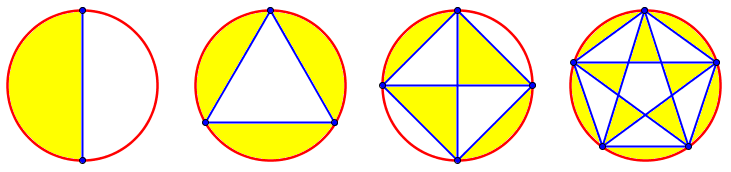Doctor Schwa answered (already knowing the answer, as we’ll see, but going along with the discovery approach):

Wow, that's quite a lot of data. Did you draw a really big circle and try to count them? I would get tired way before this. Or did you have some other method of producing this table? If so, the method you used might be a good clue for uncovering the pattern. Also, not knowing where you got these big numbers makes me wonder if there might be some small mistake, and as you already know, even if you're just off by 1 you could hide a beautiful pattern.

Noticing the Fibonacci sequence connection is a very nice start. I wouldn't have noticed that pattern at all, though Fibonacci does make some sense here because the pattern you unearthed is really subtle and hard to see.

I'll do my best to help, but you've already used such a great set of problem solving strategies I don't know if I'll be able to do much better.

Although the Fibonacci connection is interesting, we’ll see that it is wrong because the numbers aren’t quite right!

Finding a pattern in the way you count, rather than just in the numbers you get, is a key idea also discussed in the post Building Patterns and Sequences.

Here are your data one more time to refresh my memory:

dots      1   2   3   4   5   6   7   8    9   10   11   12
regions   1   2   4   8  16  30  57  88  163  230  386  456

I hope you were careful in drawing your dots not to make any special patterns with them (e.g. a regular hexagon, where several lines all pass through the point in the middle, will have fewer regions than if you used a nonregular hexagon).



Here are the next two cases, for n = 6 (twice) and n = 7. Observe that the first is a regular hexagon, and three diagonals meet in the center; in order to get the maximum number of regions, I’ve moved one point aside in the second picture, adding one region: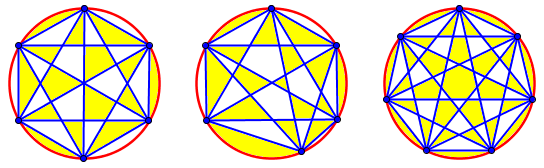So for n = 6, the correct number is not 30 but 31. (And if you were expecting 32 because of the apparent pattern, we’ll be discussing that later!) For n = 7, the regular heptagon works fine, showing 57 regions. (Go ahead and count them; I’ll wait!)

In working through this, I noticed (as I never had before) that the problem didn’t specify that we want the maximum number of regions; it’s possible that the intent was to use regular polygons. In that case, the problem that was posed is quite difficult, and in fact was only solved relatively recently! That problem is briefly discussed here (the page title is inaccurate, and you’ll have to add n to get the numbers in Daniela’s list above):

Intersections of Polygon Diagonals

But the problem Doctor Schwa is answering is one that can be solved by ordinary people, so we’ll stick with it.

### Adding one point at a time

Rather than start with numbers that we hope were counted right, it’s safer to focus on how you do the counting: the method is a clue, as he said:

The way I start working on a problem like this is to draw a picture of my own. It's clear why 1 dot does nothing new, and just leaves 1 circle. The second dot lets you draw one line, cutting the circle in half. The third dot lets you draw two lines, cutting two new regions. The fourth dot lets you draw two lines to adjacent dots, each of which cut one new region, and one line to the opposite dot, which crosses a line on the way there so it makes 2 new regions, and we get 1 + 1 + 2 = 4 new regions all told.

Each time we add an nth point, we add n – 1 new lines (in blue), and one new region for each segment of those lines: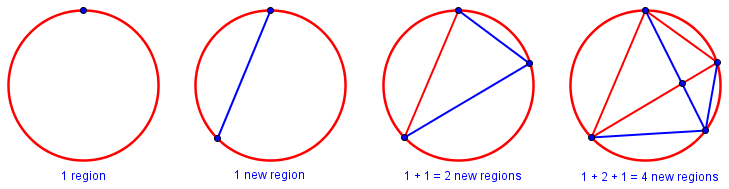For example, the last figure shows adding the 4th point, which adds 3 lines, which consist of a total of 4 segments; each segment split an existing region, so we have 4 new regions, raising the total from 4 to 8.

So then I start wondering if I can make a pattern out of that. I don't see it yet, so I scribble a couple more cases on my picture. The fifth dot connects to two adjacent dots again, making one new region each, and now there are two "opposite" dots, and I have to cross two lines on the way there, so they make a total of three new regions each, for 1 + 1 + 3 + 3 = 8 new regions.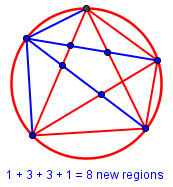So now I guess the pattern: when I add the sixth dot, I'll get 1 + 1 for the adjacent two dots I connect to, 4 + 4 for connecting to the two dots next to them, and then 5 for connecting to the opposite vertex. That means I added 15, so I get 31 regions for 6. Maybe you did the problem with a regular hexagon? Or some other kind of shape where more than two lines crossed at one point? Or you missed counting one region? Or maybe I made a mistake?  If I did, let me know.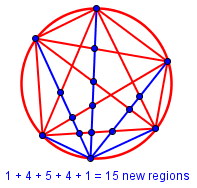Supposing I'm right so far, the pattern does get pretty complicated. I always add 1 + 1 for connecting to the two adjacent vertices, then (n-2) + (n-2) for the two next to that but it's tricky to count how many crossings I make going to the next vertices.

The way I think of it is that the number of new regions created is the number of connections I cross, plus 1. Each vertex to the left of the line I draw has to connect to each vertex to the right of the new line I draw. So when I draw that 7th dot, I get two adjacent dots giving 1 + 1, the two next to that (splitting the other 5 dots into groups of 1 and 4) gives me (4+1) + (4+1), and the remaining two (splitting the other 5 dots into groups of 2 and 3) gives me (2*3 + 1) + (2*3 + 1) so all told I get 2 + 10 + 14 = 26 new regions. So my number for 7 dots would be 31 + 26 = 57. Looks like I'm back into agreement with you!

The agreement, as we saw above, is because with 7 points (a prime number) there are no multiple intersections among diagonals for the regular heptagon.

There may well be a simple pattern where I could find, say, the answer for 100 dots without having to carefully add up everything along the way, but if there is, I don't see it now. I could try a bunch of messy algebra to express this summation formula, but that doesn't seem as if it would be fun. And even if it turned out to be fun, it doesn't sound particularly promising.

But perhaps this method of counting will let you generate a few more numbers in the list (and check, and correct some of the numbers you already have? Again, unless I'm misinterpreting the problem...) and maybe once you have a correct table you'll be able to find some pattern that I'm not seeing yet.

Actually, the pattern as described here, thought of from the right perspective, will lead to a neat solution that we’ll see in our last answer. Just to get you thinking: We start with one region; then for each new line we draw, we got one new region for each intersection we create, plus another region just for finishing the line …

### Finding a quartic equation

Last week, looking at regions formed by n chords, we saw that one method used the equality of second differences to determine that the formula was quadratic, and then we found a formula that fit algebraically. We can do the same here (now that we have used the pattern we found to confirm that we have accurate numbers):

I think the method of finite differences is very promising. In fact, just with the data we already have (if I'm right about the 31?):

1   2   4   8   16  31  57
1   2   4   8   15  26
1   2   4   7   11

where in each row I subtract the two numbers above it. I think a complete solution to the problem is not too far away at that point.

Let’s carry this out:

The next line (third differences) will be 1, 2, 3, 4, so the fourth differences are 1, 1, 1. This is enough to be reasonably confident that there is a fourth-degree formula for this sequence. So we can assume the formula is $$r = ax^4 + bx^3 + cx^2 + dx + e$$ and create five equations to solve for these five unknowns, by taking x = 1, 2, 3, 4, 5:

$$a(1)^4 + b(1)^3 + c(1)^2 + d(1) + e = 1$$

$$a(2)^4 + b(2)^3 + c(2)^2 + d(2) + e = 2$$

$$a(3)^4 + b(3)^3 + c(3)^2 + d(3) + e = 4$$

$$a(4)^4 + b(4)^3 + c(4)^2 + d(4) + e = 8$$

$$a(5)^4 + b(5)^3 + c(5)^2 + d(5) + e = 16$$

That is,

$$a + b + c + d + e = 1$$

$$16a + 8b + 4c + 2d + e = 2$$

$$81a + 27b + 9c + 3d + e = 4$$

$$256a + 64b + 16c + 4d + e = 8$$

$$625a + 125b + 25c + 5d + e = 16$$

I will not show the work, but I have actually done it, and the solution is $$a=\frac{1}{24}, b=-\frac{1}{4}, c=\frac{23}{24}, d=-\frac{3}{4}, e=1$$, so that

$$r = \frac{n^4 – 6n^3 + 23n^2 – 18n + 24}{24}$$

A post on “finite differences” has been in the works for a long time, and will hopefully be coming soon! But for more information, you can search Ask Dr. Math for the phrase.

If you'd like to talk about this problem some more, please do send me an email. This kind of problem solving is a lot of fun.

Or, if you'd like to see a more formal treatment, look at

...

## Using the pattern to make a sum

Here is the question he referred to, which was actually posted only five days earlier in 1998:

Counting Regions Formed by Chords of a Circle

Dear Doctor Math,

This is an extension of a problem we have been dealing with in class.  I would like your help in understanding it, if possible.

Let n be the number of points on the circumference of a circle, and let r be the maximum number of non-overlapping regions formed by connecting each of the n points, with a line segment, to every other point on the circle, including its two neighboring points. Let n = 1, 2, 3, 4, 5, 6 .... Find a pattern relating n and r. Find an explicit or a recursive equation for r, and prove this formula.

I would like any help you can offer with this question. It seemed straightforward, but then it just changes; and finding the formula, and being able to prove it, are not so simple. I would appreciate any help you can give me. Thank you.

Lover of Math,
Jay Shah

This one is very clearly stated. And, yes, the pattern starts out with 1, 2, 4, 8, 16, making you think the next will be 32, but it “just changes”.

Doctor Rob answered, first stating the formula that will be derived, in two forms:

The formula is r(n) = C(n,4) + C(n,2) + 1. The values for n = 1, 2, 3, ... are:

1, 2, 4, 8, 16, 31, 57, 99, 163, 256, ....

Here, C(n,k) = n!/[k!*(n - k)!]. This expression for r can also be expressed in the form:

r(n) = (n^4 - 6*n^3 + 23*n^2 - 18*n + 24)/24

That second form is just what we (well, I) found above. The first form is found most easily by a method I’ve been hinting at, which is the goal of this post. But for now, we have a method that starts with the pattern we already saw:

You can prove this by starting with n points, all connected, and adding one more point. Connecting it to its two neighbors adds 1 region each. Connecting it to their neighbors adds 1*(n - 2) + 1 regions each. Connecting it to their neighbors adds 2*(n - 3) + 1 regions each. Connecting it to their neighbors adds 3*(n - 4) + 1 each. Each line from the new point to an old vertex crosses k*(n - k - 1) lines connecting the k vertices on one side of the line to the remaining n - k - 1 vertices on the other side, and crossing m lines results in m + 1 new regions. That means the number of regions added is:

n-1
r(n + 1) - r(n) = Sum [k*(n - k - 1) + 1]
k=0

n-1
= Sum [1 + n*k - k*(k + 1)]
k=0

= n + n*n*(n - 1)/2 - n*(n - 1)*(n + 1)/3

= (n^3 - 3*n^2 + 8*n)/6

= n*(n + 1)*(n + 2)/6 - 2*n*(n + 1)/2 + 2*n

We are summing the numbers added by each new point, using a formula Doctor Schwa got close to but didn’t quite state. To carry out the summation, Doctor Rob uses known formulas for sums: $$\sum_{k=0}^{n}k = \frac{n(n+1)}{2}$$ and $$\sum_{k=0}^{n}k(k+1) = \frac{n(n+1)(n+2)}{3}$$.

Thus:

n
r(n) = r(0) + Sum [r(k) - r(k - 1)]
k=1

n
= 1 + Sum [(k - 1)*k*(k + 1)/6 - 2*(k - 1)*k/2 + 2*(k - 1)]
k=1

= 1 + (n - 1)*n*(n + 1)*(n + 2)/24
- 2*(n - 1)*n*(n + 1)/6 + 2*(n - 1)*n/2

= 1 + n*(n - 1)*(n^2 + 3*n + 2 - 8*n - 8 + 24)/24

= 1 + n*(n - 1)*(n^2 - 5*n + 18)/24

= 1 + n*(n - 1)/2 + n*(n - 1)*(n - 2)*(n - 3)/24

= 1 + C(n,2) + C(n,4)

At the end, he is reversing the process, using well-known formulas for binomial coefficients C(n,k), which is also written as $$_nC_k$$ or $${n}\choose{k}$$. It would be more natural just to produce the quartic equation we found previously.

Frankly, I’ve included this, which is a huge amount of algebra that I don’t expect anyone to follow, just to make the next answer even more satisfying.

## The elegant way

For a more direct way to the same formula, here is a question from 2003 (which was combined with an answer we looked at last time, to the other circle-region question!):

Circle Regions

We drew a circle and then put two points on the line and joined the points. The circle is divided into two parts. If there are 4 points (not evenly spaced) and they are each joined to all the other points, the circle is divided up into 8 regions.

There is a conjecture that says that if the process is repeated, with more points along the edge of the circle, not evenly spaced, then a rule for the maximum number of regions is 2 to the power of (the number of points take one) r=2^(n-1) where ^ = to the power of.

For 2,3,4,5,6 points, they follow the formula, but for 7 points, it doesn't; there are 57 regions, and if it followed the rule, there'd be 64.  Am I not counting them properly? Is there a simple way of understanding how many regions there are, because there must be a constant formula, shouldn't there be?

The “conjecture”, which is false, is that because the first terms are 1, 2, 4, 8, 16, the general term is $$r_n = 2^{n-1}$$. Sheila has seen that it doesn’t work. What does?

Doctor Anthony answered with his usual conciseness:

n points are distributed round the circumference of a circle and each point is joined to every other point by a chord of the circle.  Assuming that no three chords intersect at a point inside the circle we require the number of regions into which the circle is divided.

With no lines the circle has just one region. Now consider any collection of lines. If you draw a new line across the circle which does not cross any existing lines, then the effect is to increase the number of regions by 1. In addition, every time a new line crosses an existing line inside the circle the number of regions is increased by 1 again.

This is the pattern I pointed out above: In looking at the number of regions added by a new point on the circle, we observed that we add one region each time we cross another line, and another when we finish the line. So each interior intersection corresponds to a region, as does each line drawn. We can count them all at once, rather than point by point. For example, looking again at the case of 5 points: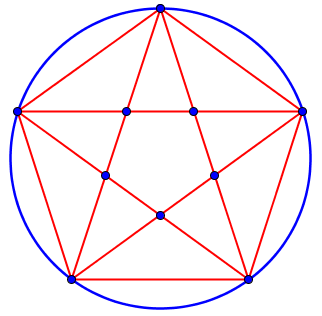There was 1 region to start, plus one each for 10 lines, plus one each for 5 intersections, for a total of $$1+10+5 = 16$$ regions.

Now, how can we calculate the numbers of lines and intersections?

So in any such arrangement

number of regions = 1 + number of lines + number of interior intersections

= 1 + C(n,2) + C(n,4)

Note that the number of lines is the number of ways 2 points can be chosen from n points. Also, the number of interior intersections is the number of quadrilaterals that can be formed from n points, since each quadrilateral produces just 1 intersection where the diagonals of the quadrilateral intersect.

That is, there is one line for each pair of points, so we count combinations of n points taken 2 at a time, $$\displaystyle {{n}\choose{2}} = \frac{n(n-1)}{2}$$. And there is one intersection for each set of four distinct points, because only one way of pairing them produces an intersection. For example, the set of four points shown here result in six different lines, but only one intersection: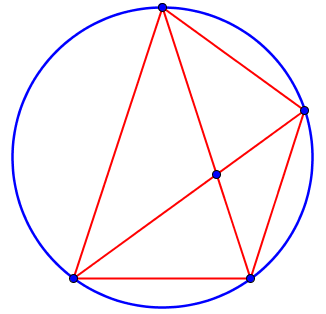So the number of intersections is the number of combinations of n points, taken 4 at a time, $$\displaystyle {{n}\choose{4}} = \frac{n(n-1)(n-2)(n-3)}{24}$$.

And we can get the quartic formula from this: $$1+{{n}\choose{2}}+{{n}\choose{4}} = 1 + \frac{n(n-1)}{2} + \frac{n(n-1)(n-2)(n-3)}{24} = \\ \frac{24+12n^2-12n+n^4-6n^3+11n^2-6n}{24} = \frac{n^4-6n^3+23n^2-18n+24}{24}$$ which we have seen before.

Examples:

n=4    Number of regions = 1 + C(4,2) + C(4,4) =  8
n=5    Number of regions = 1 + C(5,2) + C(5,4) = 16
n=6       "         "    = 1 + C(6,2) + C(6,4) = 31
n=7       "         "    = 1 + C(7,2) + C(7,4) = 57

Note that formula 2^(n-1) starts to go wrong at n=6

For an interesting question from 2001, where this week’s topic was confused with last week’s, read this:

Slicing Up a Circle

This site uses Akismet to reduce spam. Learn how your comment data is processed.